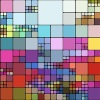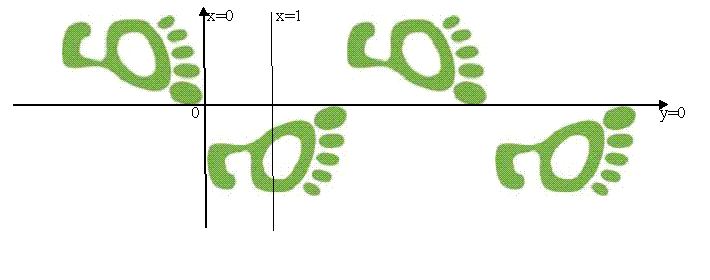#### You may also like### Sine Problem

In this 'mesh' of sine graphs, one of the graphs is the graph of the sine function. Find the equations of the other graphs to reproduce the pattern.### Squaring the Rectangle

Can you find a way to turn a rectangle into a square?### The Use of Mathematics in Computer Games

An account of how mathematics is used in computer games including geometry, vectors, transformations, 3D graphics, graph theory and simulations.

# Footprints

##### Age 16 to 18 Challenge Level:The isometries in the plane (reflections, rotations, translations and glide reflections) are transformations that preserve distances and angles.

Draw diagrams to show that all the isometries can be made up of combinations of reflections.

Complex numbers can be used to represent isometries. We write the conjugate of $z = x + iy$ as $\bar z = x- iy$.

A reflection in the imaginary axis $x=0$ is given by $\alpha (z) = -\bar z$. A reflection in the line $x=1$ is given by $\beta(z) = 2 - \bar z$. A reflection in the real axis $y=0$ is given by $\gamma (z) = \bar z$.

Find the formula for the transformation $\gamma \beta \alpha (z)$ and explain how this transformation generates the footprint frieze pattern shown in the diagram.